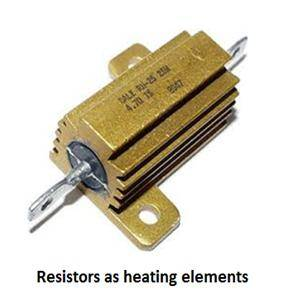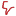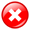Don't Miss

# Interesting Uses Of Resistors In Unique Projects

By on August 1, 2012Anything that hinders the path of current “resists” it. A resistor, just like its name suggests, resists or hinders the path of the current.Used in anelectronic circuit it acts as pure resistance of specific value.Resistance is measured in Ohms(Ω).Resistors are available with different values of resistances and power ratings.

A resistor is a basic and vital component of an electrical or electronic circuit. Wecan get different values of current in different parts of the circuit just by putting a resistor in the currents path. Its value can easily be calculated by the famous Ohm’s law.

How resistors are used in different projects:

1) Gain Control In 741 Op Amp:

An operational amplifier is used to increase the input voltage by a specific ratio known as “gain”.The gain of the operational amp is defined as output voltage divided by input voltage. The gain of 741 op amp can vary from100,000 to over 1 million. Gain requirement depends upon usage; varying from project to project. A very high gain systemcan become easily unstable and may give false output to a very small change in input due to some physical abnormality. So it is necessary to control the gain of 741 Op Amp somehow. One method to control the gain is using two resistors R1 and R2 as shown in following figure.The gain for this circuit is given by R2/R1.So the gain is being controlled by precise values of resistances here.Now using required value of gain,the 741 op-amp can be used as an audio amplifier, radio signal amplifier and many other jobs.

2) Resistors As Heating Elements:

High wattage resistances can be used as heating elements for temperatures of 70⁰C and below. The appropriate resistance wattage for this job can be 25 W or above.The high wattage ensures that when the temperature increases the resistor may not burn out.Now all that remains is providing precise voltage to the resistor. By controlling the voltage the temperature of the resistor can be controlled. For instance if a 25 W resistor is available with resistance “R”, to run it at maximum temperature the voltage is calculated byhere P=25 W.

DIY Resistors:

You can make your own resistor using different resistive materials for simple uses like current limiter or voltage divider.

Materials you need:

• Pencil
• Eureka wire
• Copper connecting wires
• LED
• Alligator clips
• Cutter

Procedure:

1. To make your own resistor you need a resistive element like graphite or some high resistive wire like eureka wire.
2. To usit as voltage divider we need the wire to be of appropriate length, for instance 6 inches. If a pencil is being used its lead should be uncovered first by using a cutter to chip off the wood of the pencil.
3. When the pencil lead is uncovered attach the extreme ends of it to a voltage source.
4. Attach the LED’s positive terminal to the end of the pencil lead connected to positive terminal of voltage source and connect LED’s negative terminal somewhere in between the two ends of the pencil lead. Now as the negative terminal of LED slides away from positive terminal of lead using alligator clip, its brightness rises as the voltage drop across it increases.
5. Now if the pencil lead is replaced with naked eureka wire of same length it will perform the same job.Likes(1)Dislikes(0)